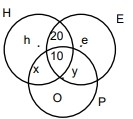# Each of 74 students in a class studies | Quantitative Aptitude Modern Maths | Set Theory

### CAT Verbal Ability

CAT 2018 – The CAT QA section has grown increasingly difficult since 2015. In order to tackle the tougher CAT Level QA questions for the CAT Exam, it is important to understand the basics of CAT. To obtain a great CAT score, make use of MBAP Free Study material with detailed solutions and video explanations. Check out MBAP free Mock test to take these questions in a test format for free.

## CAT 2018 - Slot-1 - Modern Maths - Question-3 - Each of 74 students in a class studies

Q. 3: Each of 74 students in a class studies at least one of the three subjects H, E and P. Ten students study all three subjects, while twenty study H and E, but not P. Every student who studies P also studies H or E or both. If the number of students studying H equals that studying E, then the number of students studying H is

Let the number of students who studying only H be h, only E be e, only H and P but not E be x, only E and P but not H be yGiven only P = 0 All three = 10; Studying only H and E but not P = 20 Given number of students studying H = Number of students studying E

= h + x + 20 + 10

= e + y + 20 + 10

h + x = e + y total number of students = 74 Therefore, h + x + 20 + 10 + e + y = 74

h + x + e + y = 44 h + x + h + x = 44 h + x = 22

Therefore, the number of students studying H = h + x + 20 + 10 = 22 + 20 + 10 = 52.

### Past Year Question Paper & SolutionsCounselling Session
By IIM Mentor

#### Free Material Area